## Itsc2011-hausknecht.pdf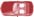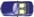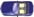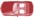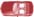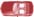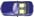To appear in Proceedings of the 14th IEEE ITS Conference (ITSC 2011), Washington DC, USA, October 2011.
Dynamic Lane Reversal in Trafﬁc Management Matthew Hausknecht, Tsz-Chiu Au, Peter Stone School of Civil and Environmental Engineering {mhauskn,chiu,pstone}@cs.utexas.edu {davidfajardo2,s.travis.waller}@gmail.com Abstract— Contraﬂow lane reversal—the reversal of lanes in order to temporarily increase the capacity of congested roads—can effectively mitigate trafﬁc congestion during rush hourand emergency evacuation. However, contraﬂow lane reversaldeployed in several cities are designed for speciﬁc trafﬁcpatterns at speciﬁc hours, and do not adapt to ﬂuctuationsin actual trafﬁc. Motivated by recent advances in autonomous An illustration of contraﬂow lane reversal (cars are driving on vehicle technology, we propose a framework for dynamic lane the right side of the road). The total capacity of the road is increased by reversal in which the lane directionality is updated quickly and approximately 50% by reversing the directionality of a middle lane.
automatically in response to instantaneous trafﬁc conditionsrecorded by trafﬁc sensors. We analyze the conditions under systems, more aggressive contraﬂow lane reversal strategies which dynamic lane reversal is effective and propose an integer can be implemented to improve trafﬁc ﬂow of a city without linear programming formulation and a bi-level programming increasing the amount of land dedicated to transportation.
formulation to compute the optimal lane reversal conﬁguration An important component of implementing dynamic lane that maximizes the trafﬁc ﬂow. In our experiments, activecontraﬂow increases network efﬁciency by 72%.
reversal is fully understanding the systemwide impact ofincreasing capacity on an individual link. We deﬁne the objective of contraﬂow as follows: given a road network,a speciﬁcation of vehicles’ locations and destinations, and Trafﬁc congestion is a major issue in today’s transportation a method for determining network efﬁciency (such as an systems. Contraﬂow lane reversal, the reversal of trafﬁc ﬂow objective function), assign a direction of ﬂow to each lane along a lane to temporarily increase the capacity of congested such that network efﬁciency is maximized. To study the roads at the expense of under-utilized ones, is a method to network effects of dynamically repurposing lanes, we cast the increase trafﬁc ﬂow without adding additional roads or lanes.
problem as a maximum multi-commodity ﬂow problem— On the left of Fig. 1, the top lanes are being more heavily a version of the maximum ﬂow problem in graph theory utilized than the bottom ones. On the right, by temporarily with multiple commodities (or goods) ﬂowing through the converting a lane to ﬂow in the opposite direction, the network. Then we propose an integer programming formula- instantaneous capacity in the left-to-right direction of the tion and a bi-level programming formulation to compute the road is increased by 50%. Contraﬂow lane reversal has been maximum ﬂow in the network. We evaluate our approaches used routinely in several cities in order to alleviate trafﬁc in grid-like transportation networks representative of many during rush hours as well as to reroute trafﬁc around certain downtown metropolitan areas where it will have the most areas such as construction sites or stadiums.
Today, contraﬂow lane reversal is used at a macro time The rest of the paper is organized as follows. In Section II, scale at rush hour or for quick evacuations from an area. In we discuss the hardware needed for implementing a dynamic both cases however, the change in ﬂow must be carefully lane reversal scheme. In Section III and IV, we analyze under planned before the event, with little or no room for dynamic what conditions dynamic lane reversal will be useful for an changes. Today’s hardware for trafﬁc monitoring is good individual road and intersection. In Section V and VI, we enough to gather real-time trafﬁc data. With the help of introduce both the macroscopic ILP trafﬁc model as well modern computerized trafﬁc control systems, it is possible as the bi-level formulation, and investigate the performance to quickly and dynamically open and close lanes or entire gains imparted by dynamically reconﬁguring lanes.
roads, or even change the directionality of lanes based onreal-time usage statistics, such that effective capacity of a road can be dynamically changed based on the demand.
A reversible lane (or contraﬂow lane) is a lane in which Rapid changes of lane directions, however, may confuse trafﬁc may travel in either direction. The common hard- human drivers. To fully utilize the potential of dynamic lane ware for creating reversible lanes is overhead trafﬁc lights reversal, we will need to rely on the upcoming availability (Fig. 2(a)). In many cities, barrier transfer machines, also of computer-aided driving systems and fully autonomous known as zipper machine, are used to relocate the moveable vehicles that will help vehicles to adjust to the rapid changes barriers such that the road in one direction can be dynami- of lane directions. With the help of computerized driving cally widened at the expense of the other (Fig. 2(b)).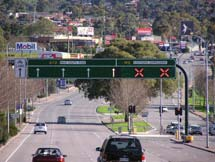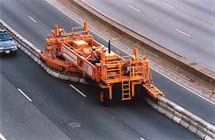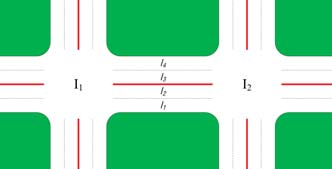Hardware for controlling contraﬂow lane.
By deﬁnition, the throughput of the road increases after the The basic requirements to support dynamic lane reversal are that the reversal has to be done quickly and safely, andthat the drivers must be notiﬁed about the change immedi- λ(L1,2) + λ(L2,1) < λ (L1,2) + λ (L2,1) ately. While it’s conceivable to devise a system that satisﬁes where λ (L1,2) = min(I1),c(L1,2) − c(l)}, and λ (L2,1) = these requirements using the hardware in Fig. 2, there is min(I2), c(L2,1) + c(l)}.
likely to be signiﬁcant cost and risk of driver confusion.
In general, lane reversal is beneﬁcial only when one of the However once most cars are controlled by computer, these directions is oversaturated while the other is undersaturated, costs and risks may be signiﬁcantly reduced by real time up- as shown in Fig. 1. Formally, we have the following theorem: dates of lane direction over wireless network communication, Theorem 1: The throughput of the road R increases after as computerized driving systems (i.e., autonomous vehicles) the reversal of a lane l ∈ La,b if and only if La,b is un- can react to the changes of lane directions much quicker than dersaturated by δa while Lb,a is oversaturated by δb, where human drivers. For example, Dresner and Stone proposed an max{c(l) − δa, 0.0} < δb.
intersection control mechanism called Autonomous Intersec- Proof Sketch. Due to space limitations, we only consider tion Management (AIM) that uses a wireless communication the case in which c(l) > δa and δb < c(l). The reversal of l protocol to enable ﬁne-grained interleaving of vehicle routes reduces the effective trafﬁc rate of Lb,a by x = c(l) − δa > 0 through an intersection . With some modiﬁcations to the while the effective trafﬁc rate of La,b increases by δb. Thus AIM protocol, autonomous vehicles can be informed about the throughput of the road increases if and only if δb > x = the current lane directions as well.
max{c(l) − δa, 0.0}.
We begin by considering, from a theoretical perspective, Analyzing the change of the intersection throughput is the effects of lane reversal on a single road. Consider a road necessary because intersections may potentially be the bot- between intersections I1 and I2. Let R be the road between tlenecks of the trafﬁc ﬂow, preventing the adjacent roads I1 and I2, L1,2 be the set of lanes from I1 to I2, and L2,1 from achieving their maximum throughput as predicted by be the set of lanes from I2 to I1. As an example, in Fig. 3, Theorem 1. Estimating the effects on intersection throughput L1,2 = {l1,l2} and L2,1 = {l3,l4}. The capacity of a lane l, theoretically, however, can be a challenging task, especially denoted by c(l), is the maximum rate at which vehicles enter when vehicles from different roads can enter the intersection the lane and is measured by the number of vehicles per hour.
at the same time. Therefore, we use empirical methods to see We assume the capacity of a set L of lanes, denoted by c(L), whether intersections can handle the increase of the incoming is the sum of the capacities of all lanes (c(L) = ∑l∈L c(l)).
trafﬁc when the directions of adjacent lanes reverse.
For simplicity, we ignore the effect of lane changing which We experiment with the intersection in Fig. 4, which has potentially reduces the capacity of L.
six lanes on each incident road. Initially, 3 lanes are incoming Assume both I1 and I2 are sources at which vehicles are lanes and 3 lanes are outgoing lanes. We set the target trafﬁc “generated” to travel along R at the target trafﬁc rates β (I1) rate of the eastbound road be 5500 vehicles per hour, the and β (I2) respectively. But the effective trafﬁc rates λ (L1,2) target trafﬁc rate of westbound road be 1100 vehicles per and λ (L2,1) at which vehicles actually enter the road are lim- hour, and the traget trafﬁc rates of both northbound and ited by the capacity of the lanes. More precisely, λ (L1,2) = southbound roads are 1650 vehicles per hour. Thus, the trafﬁc min(I1), c(L1,2)} and λ (L2,1) = min(I2),c(L2,1)}. If on the eastbound road is several times higher than other λ(L1,2) = c(λ(L1,2)), we say L1,2 is saturated. If β(I1) > roads, causing trafﬁc congestion on the eastbound road. We c(L1,2), L1,2 is oversaturated by an amount of β (I1)−c(L1,2).
check whether reversing the direction of two lanes on the Clearly, if L1,2 is oversaturated, L1,2 is saturated. L1,2 is westbound road can help to increase the throughput of the undersaturated by an amount of c(L1,2) − β (I1) if β (I1) < eastbound road as well as the intersection throughput (the c(L1,2). Clearly, if L1,2 is undersaturated, L1,2 is not saturated.
number of vehicles entering the intersection per hour). The The saturation of L2,1 is deﬁned in the same manner.
new lane conﬁguration is shown on the right side in Fig. 4.
The throughput of the road R is the sum of the effective We repeated the experiment 30 times and in each run trafﬁc rates of the lanes (i.e., λ (L1,2) + λ (L2,1)). Now con- we measured 1) the total number of vehicles entering the sider what happens if the direction of l ∈ L1,2 is reversed.
intersection during the 1-hour period, and 2) the number of The multi-commodity ﬂow problem is a generalization of the well-known max ﬂow problem in which multiplecommodities or goods ﬂow through the network, each withdifferent source and sink nodes. Modeling a road networkat the macroscopic level allows us to map the well-studiedproblem of multi-commodity ﬂow directly onto our problem Fig. 4. The reversal of two lanes on the westbound road of an intersection.
of dynamically reconﬁguring lanes. In order to solve this problem we utilize the the mathematical machinery of linear A Linear Program contains a linear function to be max- imized over a set of variables, subject to constraints. Innormal linear programs these variables are allowed to as- vehicles entering the intersection from each road during a 1- sume fractional values, but since all of our ﬂow demands hour period. The average of the number of vehicles and the are required to be integer-valued, we must approach this 95% conﬁdence intervals are shown in Table I. The results problem as an Integer Linear Program. Unfortunately, the show that the throughput of the intersection increased by 6%, multicommodity ﬂow problem has long been known to be and this is mainly due to the increase of incoming trafﬁc NP-complete when dealing with integer ﬂows, even for only from the eastbound road whose throughput is increased by 13%. Note that both increases are statistically signiﬁcant.
We deﬁne the following Integer Linear Program: Given a After lane reversal, the eastbound road’s trafﬁc rate is much graph G = {V, E}, each edge (u, v) has some integer capacity closer to the target trafﬁc rate, and this means that the c(u, v) representing the total number of lanes present on intersection successfully handled the increase of the trafﬁc that road. There are k distinct commodities (trafﬁc ﬂows) coming from the eastbound road. The lane reversal has only K1, ., Kk where each commodity Ki = (si,ti, di) has an as- minor detrimental effects on other roads, because they are sociated source si, destination ti, and demand di. Flow of undersaturated and the lane reversal does not reduce the commodity i over edge (u,v) is denoted fi(u, v).
capacity of these roads below their target trafﬁc rate.
Our objective is to ﬁnd an assignment of ﬂows which satisfy the following three constraints: The capacity con- straint, shown in Equation 2, speciﬁes that the total amountof ﬂow (in both directions) over a given (u, v) edge must While the ability to improve throughput on individual not exceed the capacity of that edge (note c(u, v) = c(v, u) in roads and at individual intersections are important proofs the undirected case). The conservation constraint, Equation of concept, the true question is whether (and how much) 3, ensures that for all non sink/source vertices the amount of dynamic lane reversal can help on a full road network. To inﬂow of a given commodity equals the amount of outﬂow.
address this question we model a road network as a graph Finally Equation 4 speciﬁes that the ﬂow of each commodity consisting of vertices and edges. Each node of the graph must meet or exceed the demand for that commodity.
represents an intersection and each edge represents a road between intersections. Additionally, each u, v edge has an (u,v)∈E ∑(fi(u,v)+ fi(v,u)) ≤ c(u,v) associated capacity c(u, v) which constrains the maximum amount of trafﬁc that road can handle (in this section we ∀i∈1.k,v∈V−{s,t}[ ∑ fi(u,v) = ∑ fi(v,w)] model intersections as having inﬁnite capacity).
Trafﬁc in the network is modeled in terms of aggregate ∀i∈1.k fi(si,w) = ∑ fi(w,ti) ≥ di demand. Speciﬁcally we consider a ﬁnite number of ﬂows, where each ﬂow has an associated source vertex, destination The goal of the ILP solver is to ﬁnd an assignment of vertex, and integer-valued demand. For example, a parking directionality to each lane which maximizes the objective garage at a mall could be a source, and a bridge at the function (Equation 5) subject to the constraints speciﬁed edge of the network could be a destination. The demand above. This is done by assigning integer values to individual represents the instantaneous number of vehicles that want to ﬂows. We choose to use the maximum multi-commodity travel between these two points. Vehicles may take any path objective function in which the objective is to reconﬁgure between the source and destination so long as they do not the network to maximize the sum of all commodity ﬂows: violate capacity constraints of roads. We seek to determine 1) whether or not the lanes of a given road network can be maximize ∑ ∑ fi(si,w) dynamically reconﬁgured in order to accommodate a given set of trafﬁc ﬂows and 2) what is the maximum demand a While multiple possible objective functions could meet our criterion of ﬁnding a lane conﬁguration capable of handling a given set of ﬂows, we choose the maximum multicommodityobjective because it forces the ILP solver not only to satisfy the ﬂow demands, but also to ﬁnd the absolute maximum amount of trafﬁc a network can handle. In contrast, thefollowing section explores an alternative, least-cost objectivefunction.
C. Bi-Level Programming Formulation The multicommodity ﬂow formulation of our problem is convenient in that its solution—the maximum ﬂow—is unique and independent of vehicles’ behavior. However it ignores the fact that drivers are self-interested—they are A example generated graph with two incident S, T ﬂows. Thick concerned about their own travel times and have no incentive lines represents highways, medium lines represent arterial roads, and thin to cooperate to achieve the maximum ﬂow of the network.
Therefore, we consider an alternative formulation for the adaptive capacity problem using a bi-level approach, where ROAD TYPES COMPOSING THE RANDOMLY GENERATED NETWORKS.
the objective is to set link capacities such that, as ﬂows are determined by User Equilibrium behavior, the total system travel time, i.e. the sum of the travel times of all users, is minimized. By modeling the route choice user behavior as a travel cost minimization, we can more accuratelycharacterize the behavior of users in a trafﬁc context. The allocated to a link (i, j) that is taken from the reverse link mathematical formulation is shown in Equations 6–8. The ( j,i). The ﬁtness function used is the total system travel upper level problem includes the allocation of capacity x to time in the underlying UE problem given a decision vector each of the links, while the lower level problem is the classic User Equilibrium model presented by Wardrop : min f (x) s.t. − ci j < xi j < c ji In this section we use the ILP solver to empirically compare different trafﬁc management systems – those which can reverse lane directions quickly and those which can reverse slowly or not at all. We hypothesize that trafﬁc ∑vodji bod(i) ∀i,od.vij ≥ 0 ∀i, j (8) managers which have the ability to quickly reverse the ﬂow of trafﬁc along lanes will achieve higher throughputs than where c is the capacity vector, vod is the ﬂow vector for each OD, x is the dynamic lane capacity allocation vector, t fis the free ﬂow speed vector, and α and β are parameters, and bod(i) is equal to the node supply/demand for each OD pair We automatically generate graphs qualitatively similar to od. As bi-level problems such as this are difﬁcult to solve downtown regions of many cities. Each graph takes the form exactly, we present a Genetic Algorithms based solution of a connected planar grid. To determine the capacities for each road, we randomly select from one of the three roadtypes shown in Table II with the associated probabilities.
D. Genetic Algorithm Solution Method Flows are generated by selecting a random source, sink Genetic algorithms (GAs) is a global search heuristic that vertex pair from the graph. An example road network is uses techniques inspired by evolutionary biology (, ).
GAs are based on the assumption that the best solution isfound in regions of solution space having a high proportion of good solutions. GAs explore the solution domain to To measure the performance difference between trafﬁc identify the promising region and then search the promising control systems with the ability to quickly reverse lanes (such regions more intensely. GAs start with a population of ran- as the AIM protocol) and those that can only slowly reverse domly regenerated individuals that evolves with generations lanes (such as zipper machines), we evaluate each trafﬁc based on the principle of survival of the ﬁttest. Unlike management system for 10 hours. Each hour a new set of classical methods, GAs work with a population of points.
random ﬂows is spawned, and it is the job of the trafﬁc Therefore, the chances of getting trapped at local optima manager to accommodate this trafﬁc as well as possible by are reduced. Moreover, many variations of GAs are suitable reversing lane directionality. However not all trafﬁc managers for handling complex problems involving discontinuities, will be able to reverse lanes every hour. Trafﬁc management disjoint feasible spaces, and noisy function evaluation , systems differ in their reconﬁguration period – the amount , . In our formulation, a gene represents the capacity of time that must elapse between lane reconﬁgurations. For example, a system with reconﬁguration period of 2 will reconﬁgure lane directions every other hour while a system with reconﬁguration period of 5 will only reconﬁgure everyﬁfth hour. If a trafﬁc manager is unable to reconﬁgure lanes for a given hour, the network throughput is computed by ﬁnding the maximum multicommodity ﬂow over the cur- rent lane conﬁguration (e.g. directed multicommodity ﬂow) without allowing the directions of any lanes to change. On the other hand, if a trafﬁc manager is able to reconﬁgure lanes for a given hour, we compute throughput by ﬁnding the maximum multicommodity ﬂow over the network consistingentirely of undirected edges. This gives managers with lowreconﬁguration period the ability to more fully adapt to An example run proceeds as follows: we ﬁrst randomly Total network throughput (vehicles) as a function of DLR period.
generate a road network using the procedure described above.
A reconﬁguration period of 1 means that the network was reconﬁguredeach timestep; 2 means every other timestep; ∞ means the network was Initially this network is conﬁgured in a balanced manner never reconﬁgured (always in balanced, directed conﬁguration). Error bars in which the capacity of each road is divided as evenly as denote 95% conﬁdence intervals. Period 1,2,3 are statistically signiﬁcant possible between lanes ﬂowing in one direction and those with respect to period 0 as well as each other.
in the opposite. Next, random ﬂows are generated and the technology which can adapt quickly to changing trafﬁc ﬂows ILP solver is used to compute the network throughput for that hour either on the current directed conﬁguration (if themanager cannot reconﬁgure this hour) or on the undirected conﬁguration (if the manager can reconﬁgure this hour). Any This section shows preliminary results of the implemen- changes made to the directionality of lanes carry over into tation of the bi-level formulation presented in Section V-C.
the next hour when new ﬂows are generated.
The objective of these numerical results is to show that the In our experiments we evaluated networks of size 100 algorithm is implementable for modest sized problems.
(10x10) for 10 hours. Each hour contained a set of 4 We solve a problem on a 10x10 grid network, where the randomly generated ﬂows. Demands for these ﬂows were number of lanes varies for each link, the capacity is 1800 set to 0 to ensure that the ILP solver would both reach a vehicles/hour/lane, α = 0.1, β = 4, and t f is 25 mph, 35 mph valid solution and maximize the achievable throughput.1 The and 55 mph depending on the number of available lanes.
throughput of each trafﬁc manager was evaluated at each For the GA, the size of the population and the number of timestep and the total throughput for a trafﬁc manager with a generations were both set to 30, the mutation probability was given reconﬁguration period was the sum of the throughputs set to 0.002, and the cross-over probability was set to 0.75.
it achieved over all 10 hours. We evaluated the performance Based on these parameters, the model was solved for each of each trafﬁc manager over 34 different networks (34 trials) of 10 time periods. In order to compare the behavior of the GA based solution method with the ILP solver, we examine Figure 6 shows the total network throughput achieved by the resulting lane conﬁgurations after each time period and trafﬁc managers with different reconﬁguration periods. The calculate the correlation coefﬁcient of the solution vectors results show a signiﬁcant increase in performance of trafﬁc for the ILP and GA. The correlations in lane conﬁguration managers who have some form of lane reconﬁguration in for each of the 10 hours are shown in Table III. As shown by comparison to no lane reconﬁguration. This is not surpris- the resulting correlation coefﬁcients, the two solution vectors ing since the beneﬁts of contraﬂow are well established.
are quite different, which conforms to the idea that increased However, we seek to address the question of how much accountability in the driver route choice response process performance increase is bestowed by frequent rather than will lead to signiﬁcantly different solution strategies.
infrequent lane reconﬁguration. Infrequent reconﬁguration While the bi-level and ILP solutions show signiﬁcant (reconﬁguration period = 3,4,5) shows only modest im- differences, we expect that both solutions are valid under provements over the static conﬁguration, approximately 11% the different assumptions each model makes. Because of the throughput gain. However, decreasing our reconﬁguration pe- highly redundant grid based network topology, we expect that riod to 2, we see a 32% performance gain over the static case.
(source, destination) demands may be satisﬁed by multiple Finally, the fully dynamic reconﬁguration period 1 trafﬁc possible paths. We hypothesize that the discrepancy in the manager provides a 72% increase in throughput compared solutions results from different paths being utilized for the to the static network. This trend suggests that the large same set of demands. However, while both models are ﬁnd- gains in trafﬁc efﬁciency are achievable with reconﬁguration ing valid solutions given their constraints, we should expectthe bi-level formulation’s solution to be more realistic in an 1Incorporating non-zero demands is straightforward to implement but could have resulted in the ILP solver being unable to ﬁnd valid solutions actual trafﬁc network because the bi-level model incorporates many aspects of real trafﬁc such as congestion, equilibrium agenda includes designing car control and intersection con- CORRELATION COEFFICIENTS OF ILP AND GA SOLUTION VECTORS trol policies for dynamic lane reversal.
Acknowledgments. This work has taken place in part in theLearning Agents Research Group (LARG) at UT Austin. LARG and speed limits. On the other hand, the bi-level model can research is supported in part by NSF (IIS-0917122), ONR (N00014- guarantee only approximate solutions while the ILP solver 09-1-0658), and the FHWA (DTFH61-07-H-00030).
 K. Dresner and P. Stone, “A multiagent approach to autonomous Contraﬂow has been studied extensively in scenarios in- intersection management,” Journal of Artiﬁcial Intelligence Research(JAIR), March 2008.
volving emergency evacuations from speciﬁc locations ,  S. Even, A. Itai, and A. Shamir, “On the complexity of timetable and , , , . For example, early work by Zhou multicommodity ﬂow problems,” SIAM J. Comput., vol. 5, no. 4, pp.
et al.  and Xue  developed contraﬂow lane control  J. Wardrop, “Road paper. some theoretical aspects of road trafﬁc systems for managing the George Massey tunnel. These research.” in ICE Proceedings: Engineering Divisions, vol. 1, no. 3.
systems predicted trafﬁc demand and reversed lanes in order Ice Virtual Library, 1952, pp. 325–362.
to reduce congestion and delays. In contrast, our approach is  H. Adeli and S. Hung, Machine learning: neural networks, genetic algorithms, and fuzzy systems.
applicable to any road network which can be represented as  H. Adeli and S. Kumar, “Distributed genetic algorithm for structural graph. Heuristic solutions to contraﬂow for emergency evac- optimization,” Journal of Aerospace Engineering, vol. 8, no. 3, pp.
uations were considered by several authors , , .
 C. Fonseca and P. Fleming, “An overview of evolutionary algorithms in Particularly, Kim et al.  examine evacuation scenarios multiobjective optimization,” Evolutionary computation, vol. 3, no. 1, with multiple sources. However, their model does not allow individual lanes to be reversed and instead requires that each  K. Sarma and H. Adeli, “Fuzzy genetic algorithm for optimization of steel structures,” Journal of Structural Engineering, vol. 126, 2000.
road be converted into a single direction of ﬂow. Cova and  ——, “Fuzzy discrete multicriteria cost optimization of steel struc- Johnson  present a model for identifying optimal lane- tures,” Journal of Structural Engineering, vol. 126, p. 1339, 2000.
based evacuation routing plans for road networks using a  W. Zhou, P. Livolsi, E. Miska, H. Zhang, J. Wu, and D. Yang, “An intelligent trafﬁc responsive contraﬂow lane control system,” in mixed integer programming solver. Unlike most models, they Proceedings of the IEEE ehicle Navigation and Information Systems consider the effects of intersection merging on overall trafﬁc Conference, 1993, pp. 174–181.
and seek to ﬁnd routing solutions which avoid crossing ﬂows  D. Xue and Z. Dong, “An intelligent contraﬂow control method for real-time optimal trafﬁc scheduling using artiﬁcial neural network, of trafﬁc. However, this work considers only evacuations fuzzy pattern recognition, and optimization,” IEEE Transactions on on static road networks and does not attempt to reverse or Control Systems technology, vol. 8, no. 1, pp. 183–191, 2000.
reconﬁgure lanes. In contrast to these authors, we do not  M. Jha, K. Moore, and B. Pashaie, “Emergency evacuation planning with microscopic trafﬁc simulation,” Transportation Research Record: take the perspective of an evacuation and instead focus on Journal of the Transportation Research Board, no. 1886, pp. 40–48, contraﬂow to optimize every-day trafﬁc efﬁciency.
 H. Tuydes and A. Ziliaskopoulos, “Network re-design to optimize evacuation contraﬂow,” Proc. 83rd Annual Meeting of the Transporta-tion Research Board, Tech. Rep. 04-4715, 2004.
In this paper, we propose a framework of dynamic lane  G. Theodoulou, “Contraﬂow evacuation on the westbound i-10 out of reversal—directionality of lanes are updated quickly and the city of new orleans,” Master’s thesis, Louisiana State University, automatically in response to instantaneous trafﬁc conditions  G. Theodoulou and B. Wolshon, “Alternative methods to increase recorded by trafﬁc sensors. Recent advances in robotic the effectiveness of freeway contraﬂow evacuation,” Transportation research leads us to believe that cars will be the key to Research Record: Journal of the Transportation Research Board, no.
unlocking the full potential of dynamic lane reversal schemes  Q. Lu, B. George, and S. Shekhar, “Capacity constrained routing since they eliminate human errors. Here we established algorithms for evacuation planning: A summary of results,” in Proc. theoretical conditions under which contraﬂow lane reversal of 9th International Symposium on Spatial and Temporal Databases can increase the efﬁciency of a road, and showed empirically (SSTD’05), 2005, pp. 22–24.
 S. Kim and S. Shekhar, “Contraﬂow network reconﬁguration for that contraﬂow has beneﬁcial effects on intersection through- evacuation planning: A summary of results,” in Proc. 13th ACM put. We then formulated the problem of contraﬂow lane International Symposium on Advances in Geographic Information reversal as a multicommodity ﬂow problem, and described Systems (GIS’05), 2005, pp. 250–259.
 B. Wolshon, “One-way-out: Contraﬂow freeway operation for hurri- how to compute the optimal direction for each lane by an cane evacuation,” Natural Hazards Review, vol. 2, no. 3, pp. 105–112, integer linear programming solver. Our experimental results indicate that a) contraﬂow in any form provides increased  S. Kim, S. Shekhar, and M. Min, “Contraﬂow transportation network reconﬁguration for evacuation route planning,” IEEE Transactions on network efﬁciency and b) dynamic (fast-paced) lane reversals Knowledge and Data Engineering, vol. 20, no. 8, pp. 1115–1129, provide large performance gains (72% in our case) over slower pace lane reversal schemes. We also introduced a bi-  T. J. Cova and J. P. Johnson, “A network ﬂow model for lane- based evacuation routing,” Transportation Research Part A: Policy and level programming formulation which accounts for link level Practice, vol. 37, pp. 579–604, 2003.
congestion and user equilibrium conditions, and comparedthe results generated by Genetic Algorithms to solutions inthe multicommodity ﬂow formulation. Our ongoing research

Source: http://cse.unist.ac.kr/~chiu/papers/Hausknecht11Dynamic.pdf

### Draft

Hull and East Riding Prescribing Committee Prescribing Framework for Sirolimus (RAPAMUNE) Post Renal Transplant Patients Name:………………………… Unit Number: ……………… Patients Address:………………………(Use addressograph sticker) G.P’s Name:……………………………………………………….……. Communication We agree to treat

### hairprolaserbrush.com

Rolf HOFFMANN TrichoScan: combining epiluminescence microscopy with digital image analysis for the measurement of hair growth in vivo Hair loss or hair thinning is a common complaint in clinical dermatology,and patients seeking advice for hair loss are not necessarily bald. Also theeffects of treatment attempts are hard to measure. Consequently, there is aneed for a sensitive tool to monit